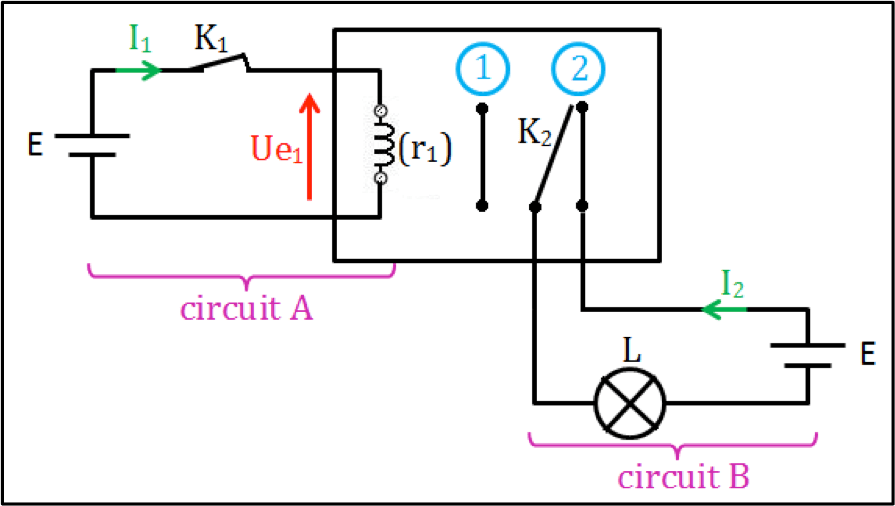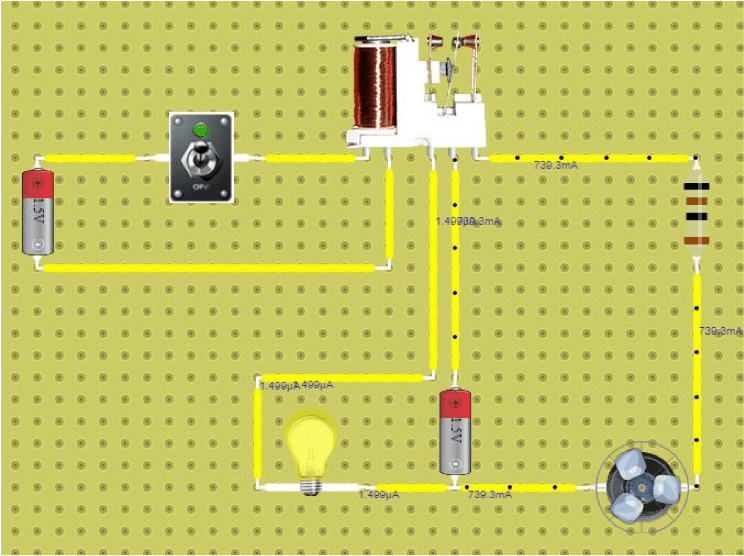MAKING A CIRCUIT USING A RELAY

# MAKING A CIRCUIT USING A RELAY

1. INTRODUCTION
An electromagnetic relay in its switching principle is a mechanical switch which is operated with a low-power DC voltage. The switch part is used to control high power circuits.
2. SPDT RelayRelay designThe SPDT relay is mainly made up of :1. Movable armature
2. Control coil
3. Switch contact points
4. Coil terminals
5. Common terminal

Description

RELAY SPDT (single pole double throw) has a total of five terminals Out of these two are the coil terminals. A common terminal is also included which connects to either of two others. When a voltage is applied to the control circuit, the coil traversed by a current will create an electromagnetic field; this latter is capable of moving a metal element called movable armature.

3. Experimentation*.Objective:
Make circuit by using a relay in the DCAClab simulator.*. Equipment:
• Battery (Electromotive Force E = 1.5 v , Internal resistance r = 1 Ω)
• Switch K1
• Coil (r1 = 1Ω)
• Relay Switch
• Lamp (r2 = 1Ω)
• Fan

*. Experiment 1: order a single circuit

• Experimental mounting• Interpretation
• Level 1
1st case: Relay at rest
The switch K1 open ( I1 = 0  ) ⇒K2 at the position (1), consequently  the circuit B is open : I2 = O , so the lamp L is off .
2nd case: Relay at work
The switch K1 is closed ( I1 ≠ 0 )⇒Kat the position (2), consequently  the circuit B is close : I2 ≠ 0 , so the lamp L is on .
• Level 2_ calculating the intensity of the current I1:Ohm’s law: Ue= r1 I= E – r I1
So  I1  = E/ (r1  + r)  = 0.04838A ≈ 48.38 mA

_ calculating the power P1:

P1 = E I1 = 1.5 x 0.04838 = 0.07257 w

_ Value of the intensity I2:

By using the characteristics of the lamp we find I2 = 1.456 A

_ calculating the power P2:

P2 = E I2 = 1.5 x 1.456 = 2.184 w

Conclusion:

The relay can be used to control a high power circuit by a low power circuit.

• Experimental verification using the DCAClab simulator*. Experiment 2 : order 2 circuits

• Experimental mounting1st case: Relay at restThe switch K1 is open ⇒K2 at the position (1), consequently:
• The circuit B is closed, so the lamp is on.
• The circuit C is open, so the fan is off.
• Experimental verification using the DCAClab simulator2nd case : Relay at workThe switch K1 is closed⇒K2 at the position (2) , consequently  :
• The circuit B is open, so the lamp is off.
• The circuit C is closed, so the fan is on.
• Experimental verification using the DCAClab simulatorthe end.

•
•
•
•
•
•

## 5 Replies to “MAKING A CIRCUIT USING A RELAY”

1.Ashley says:

Its nice to play with it. The UI makes me feel like i’m using an actual Relay unit in a lab.

2.Lizzette Mangubat says:

Keep up the great work guyz.

3.Bodybuilding Website says:

Hello! Do you use Twitter? I’d like to follow you
if that would be okay. I’m definitely enjoying your blog
and look forward to new posts.

1.Samir Sabri says:

Hi 🙂 Yes, we use Twitter, our account is @DCACLab , next release will have an improved Oscilloscope, we will be tweeting about once its released!